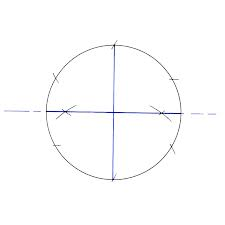## Dividing a Circle into Four or Eight Equal Parts Assignment Help

Assignment Help: >> Circle and Curves - Dividing a Circle into Four or Eight Equal Parts

Dividing a Circle into Four or Eight Equal Parts:

To draw an outline of the lid, displayed in diagram (a), it is essential to divide the circle into eight equal parts in the following way. First draw two mutually perpendicular axes, horizontal and vertical in diagram (b) to intersect at point O. From the point O like the center, describe a circle on that the eight holes are to be spaced. The point of intersection 1, 3, 5 and 7 of the circle along with the horizontal and vertical axes will divide the circle into four equal parts. Points 2, 4 6 and 8 are obtained along with the aid of a compass via bisecting the angle 103, 305, 507 and 701, respectively. A circle can be divided into four and eight equal parts also with means of a 45o - 45o triangle in diagram(c). When dividing a circle into four (4) or eight (8) equal parts along with the aid of a triangle, the lines drawn along its hypotenuse must pass with by the center of the circle.#### Assured A++ Grade

Get guaranteed satisfaction & time on delivery in every assignment order you paid with us! We ensure premium quality solution document along with free turntin report!

All rights reserved! Copyrights ©2019-2020 ExpertsMind IT Educational Pvt Ltd